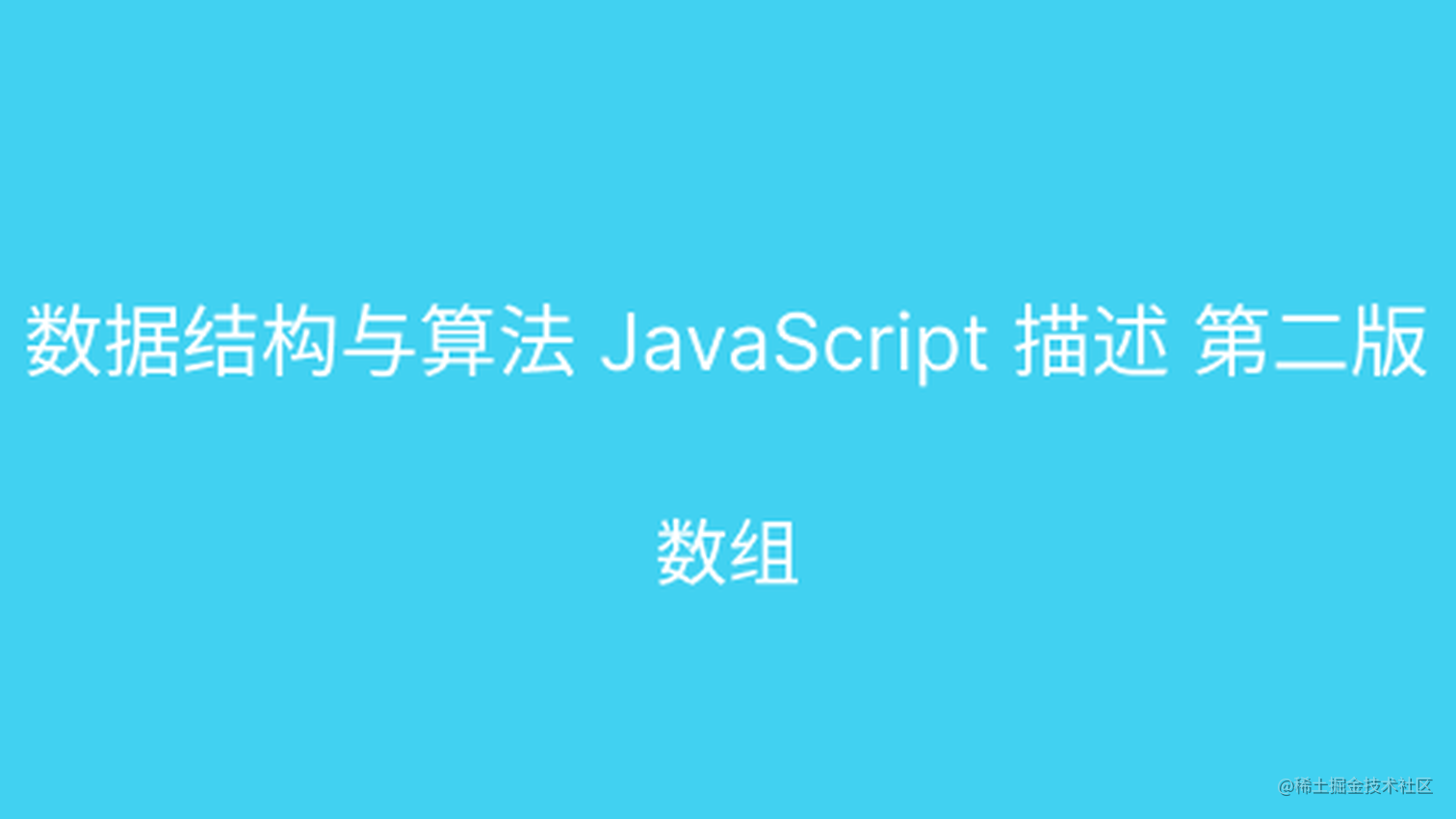# 阅读《数据结构与算法 JavaScript 描述 第二版》之数组## 概述

JS 数组无需自己实现，但需要掌握 JS 内置方法。JS 本身提供了众多的 API 操作数组，在 ES5+ 之后增了更多的数组的操作方法，对第三方库如 lodash 等函数库依赖越来越少了。

## JS 数组定义

• 标准定义：存储元素的线性集合。
• JS 数组是对象，存储元素，是非线性
• 通过索引访问

## 使用数组

### 创建数组

``````let numbers = []; // 初始化
let nums = [1, 2, 3]; // 初始化有元素
let ns = new Array(); // 使用构造函数
let ns10 = new Array(10); // 指定长度

### 读写数组

• 使用`下标`读取
• 使用 `=` 访问
``````let arr = [1, 2, 3];
let arr1 = arr
arr = 123

### 字符串生成数组

``````let date = '2022-09-10';
let dateArr = date.split('-'); // [ '2022', '09', '10' ]

### 对数组的整体性操作

• 数组的浅复制 （引用地址相同）
• 数组的深复制（引用地址不相同）

## 存取函数

### 查找元素

• `indexOf/lastIndexOf` 查找元素
``````let a = ['a', 'b', 'c']
let aIndex = a.indexOf("b") // 1

let b = ['a1', 'b1', 'c1', 'a1']
let a1Index = b.indexOf('a1') // 3
let a1LastIndex = b.lastIndexOf('a1') // 0

### 数组的字符串表示方法

• join 拼接成字符串
• toString 内置支持变化成字符串方法
``````let b  = ['a1', 'b1', 'c1', 'a1']
b.join("-") // 'a1-b1-c1-a1'
b.toString() // 'a1,b1,c1,a1'

### 由已有数组创建新数组

• concat
• splice
``````let c = ['a', 'b']
c.concat('c') // [ 'a', 'b', 'c' ]

let d = ['a', 'c']
d.splice(1, 0, 'b') // d: [ 'a', 'b', 'c' ]

## 可变函数

### 为数组添加元素

• push
• unshift
``````let e = ['a', 'b']
e.push('c') // 返回长度 3， e 数组的值是: [ 'a', 'b', 'c' ]
e.unshift('c') // 返回长度是4， e 数组的值是：[ 'c', 'a', 'b', 'c' ]

### 从数组中删除元素

• pop
• shift
``````let a = [1,3,5,7]
a.pop() // 返回是 7， 数组 a: [ 1, 3, 5 ]

let b = [1,3,5,7]
b.shift() // 返回是 1， 数组 a: [ 3, 5, 7 ]

### 从数组中间位置添加和删除元素

• splice
``````let b = [1,2,3,4,5]
b.splice(2,0, 'this is splice add str'); // b [ 1, 2, 'this is splice add str', 3, 4, 5 ]

• reserve
• sort

## 迭代方法

### 不生成新数组的迭代器方法

• forEach
• every
• some
• reduce/reduceRight
• map/filter
``````let b = [1,2,3,4,5]
b.forEach(a => {console.log(a)}) // 1 2 3 4 5

let b = [1,2,3,4,5] // false
b.some((e) => e === 1) // true
b.reduce((a, c) => a + c) // 15
b.reduceRight((a, c) => a + c) // 15

let a = [1, 2, 3, 4, 5]
a.map((aa) => aa + 1) // [ 2, 3, 4, 5, 6 ]

let afterFilter = a.filter((aa) => aa != 3) // [ 1, 2, 4, 5 ]

### 生成新数组的迭代器方法

• map 生成一个新的数组
• filter 生成符合条件的新数组

## 二维和多维数组

### 创建二维数组

• 数组 + for
``````let arr = []
let rows = 5;

for (let i = 0; i < rows; ++i) {
arr[i] = []
}
// arr [ [], [], [], [], [] ]

• 按照列访问
• 按照行访问

### 参差不齐的数组

``````let arr = [[1, 2], [3, 4, 5], [6, 7, 8]];

for (let i = 0; i < arr.length; ++i) {
for (let j = 0; j < arr.length; ++j) {
console.log("val:", arr[i][j])
}
}

## 对象数组

``````function Point(x, y) {
this.x = x;
this.y = y;
}

let pArr = []

pArr.push(new Point(1, 2))
pArr.push(new Point(3, 4))

## 对象中的数组

``````function DateStore() {
this.store = []
}

this.store.push(content)
}

let ax = new DateStore()

console.log(ax.store) // ['flex']

## 小结

• 数组在数据结构中占据重要位置。
• 数组作为 JavaScript 的引用类型，在于后端交互时常用数据类型，熟悉相关的操作十分重要。
• 数组是 JavaScript 内置类型支持，与 Java 等其他语言定义的数组有所区别。
• 熟练 JavaScript 数组的各种函数操作。
• 了解 JavaScript 多维数组。